# 6th-8th Grade Math: The Coordinate Graph Chapter Exam

Exam Instructions:

Choose your answers to the questions and click 'Next' to see the next set of questions. You can skip questions if you would like and come back to them later with the yellow "Go To First Skipped Question" button. When you have completed the practice exam, a green submit button will appear. Click it to see your results. Good luck!

### Page 1

#### Question 2 2. The following graph displays which point?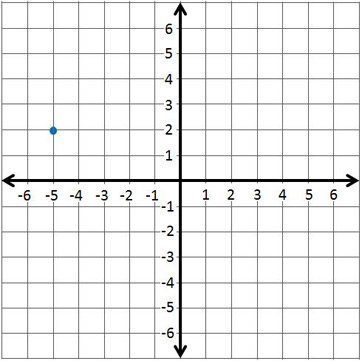#### Question 5 5. What are the coordinates of this point?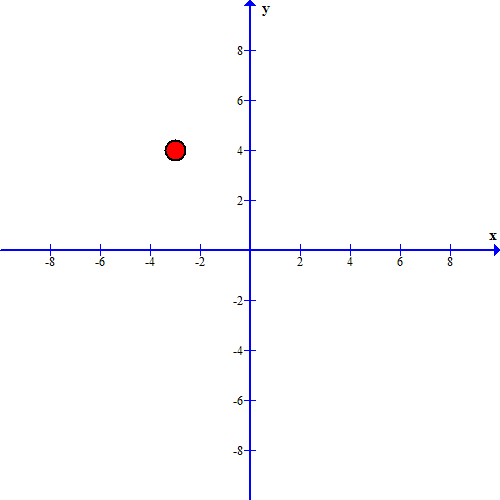### Page 2

#### Question 6 6. In which quadrant is the point (-2, 5)?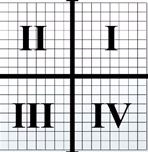#### Question 7 7. The following graph displays which point?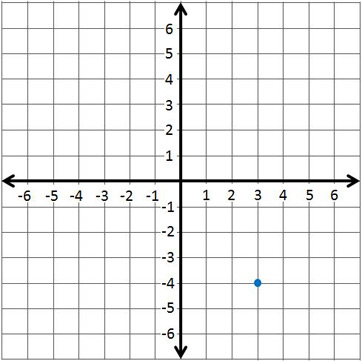#### Question 9 9. Use the number line to solve -4 + 6 - 3 + 7.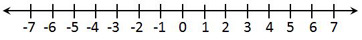#### Question 10 10. What number does the point on this line represent?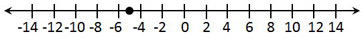### Page 3

#### Question 14 14. Use the number line to solve 9 - 13 + 7 - 3.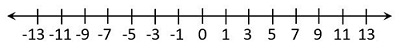#### Question 15 15. Use the number line to solve -5 - 2 + 3.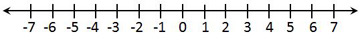### Page 4

#### Question 16 16. The following graph displays which point?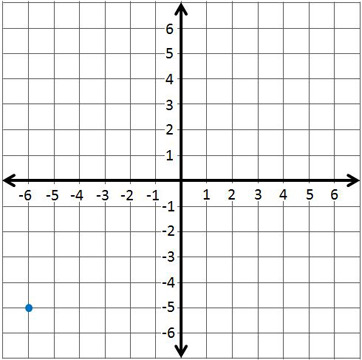#### Question 18 18. In which quadrant is the point (3, 6)?#### 6th-8th Grade Math: The Coordinate Graph Chapter Exam Instructions

Choose your answers to the questions and click 'Next' to see the next set of questions. You can skip questions if you would like and come back to them later with the yellow "Go To First Skipped Question" button. When you have completed the practice exam, a green submit button will appear. Click it to see your results. Good luck!

Support